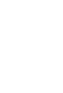## Questions

Answer all the questions in this section in the spaces provided

1. The diagram below shows a pulley system, a mass of 10kg is raised 2m by an effort of 80N.1. Calculate the distance moved by effort. 2mks
2. How much potential energy does the load gain? 2mks
3. How much work is done by the effort? 2mrks
4. What is the efficiency of these pulleys? 2mrks
5. The efficiency of a pulley system is always less than 100%. State two reasons. (2 marks)
2.
1. State the principle of conservation of Linear momentum. (1mk)
2. Calculate the recoil velocity of a gun of mass 0.4kg which fires a bullet of mass 0.090kg at a velocity of 600m/s. (3mks)
3.
1. State two factors which affect frictional force of a body. (2mrks)
2. Suggest any two ways in which friction can be minimized. (2mks)
3. State two advantages of friction. (2mks)
4. A trolley of mass 0.5kg moving with a velocity of 1.2ms-1collides in elastically with a second trolley of mass 1.5kg moving in the same direction with a velocity of 0.2ms-1.
1. What is an inelastic collision? (2mk)
2. Determine the velocity of the trolleys after collision. (2mks)
3. Define the following terms as used in refraction
1. Total internal reflection (1mrks)
2. Critical angle ( 1mrks)
4. State the two law of fraction of light. (2mks)
5.
1. Differentiate the following terms, (1maks)
1. Distance and displacement
2. Speed and velocity (1 marks)
3. Acceleration and deceleration. (1 marks)
2. An object at rest is dropped from a height of 80m.
1. Sketch a velocity-time graph for the object. (2marks)
2. Determine how long it takes to reach the ground (3 marks)
3. Determine the velocity as it hits the ground. (3marks)
6.
1. State the basic law of electrostatics. (1mark)
2. The figure below shows an arrangement which may be used to charge a capacitor of capacitance 50µF and then to connect it to a capacitor of capacitance 20µF.The switch S is first placed at position A, so that the capacitor C, is connected to the 12V dc supply.
Calculate the charge stored in the capacitor. (3marks)
7.
1. Study the figure below and use it to answer the questions below it.Determine the:
1. Current flowing through the ammeter. (3marks)
2. potential difference between X and Y (2marks)
2. State two factors that affect electrical resistance of a conductor. (2marks)

## Marking Scheme

1.
1. V.R = 2 = Distance moved by loadDistance moved by effort
2 = x2✔1
2x = 2m
x = 1m✔1
2. P.E = mgh
= 10 x 10 x 2
= 200J✔1
3. Work input = Effort x Effort distance
= 80N x 1m
= 80 Joules✔1
4. MAVR x 100% = efficiency✔1
100802 x 100% = 500080
Eff = 62.5%✔1
5.
• Friction between moving parts of the pulley system / friction1
• Weight of the movable part of pulley // work done in lifting the moving parts of pulley system
2.
1. The sum of kinetic energy and potential energy of a system is constant
Or
Energy can neither be created nor destroyed but can be transformed from one form to another
Any 1 mark
• The sum of total linear momentum before and after collision is constant.
The momentum before collision = ∑Momentum after collision. √1
m1u1 + m2u2 = m1v1 + m2v2
0.4 x 0 + 0.090 = 0.4 x v1 + 0.09 x 600 √1
0 = 0.4v1 + 54√1
V1 = -135m/s
3.
1.
• Nature of the surface√1
• Weight (Reaction) √1
2.
• Lubrication
• Rollers
• Air cushioning
4.
1. A collision in which objects combine / fuse, losing kinetic energy in the process (1mrk)
2. Final momentum = Initial momentum (3mks)
(0.5 + 1.5 ) V = (0.5 x 1.2) + (1.5 x 0.2)
2.0V = 0.6 + 0.3
2.0V = 0.9
V = 0.45 m/s
3.
1. Phenomena that occurs when angle of incidence exceeds the critical angle thus light gets reflected internally within the denser medium.
2. Critical angle is the angle of incidence in the denser medium for which the angle of refraction in the less dense medium is 90o.
4.
1. the incident rays, the refracted ray and the normal at the point of incidence all lie in the same plane.
2. the ratio of the sine of the angle of incidence to the sine of the angle of refraction is a constant for a given pair of media.
5.
1.
1. Distance is a scalar quantity while displacement is a vector quantity.
2. Speed is the rate of change of distance with time while velocity is the rate of change in displacement with
3. Acceleration is the rate of change in velocity with time while deceleration is the rate at which velocity decreases with time.
2.
1.2. S = ut + ½ at2
80 = ½ x 10t2
T2 = 16
T = 45
3. V2 = u2 + 2as
= 2 x 10x 80
= 1600
V = 40ms-1
6.
1.
1. Unlike charges attract like charges repel.
2. Charge — Voltage x Capacitance√
Q=CV
= 12X50µF√1
= 600µC or 6 x 10-4C√1

• ✔ To read offline at any time.
• ✔ To Print at your convenience
• ✔ Share Easily with Friends / Students